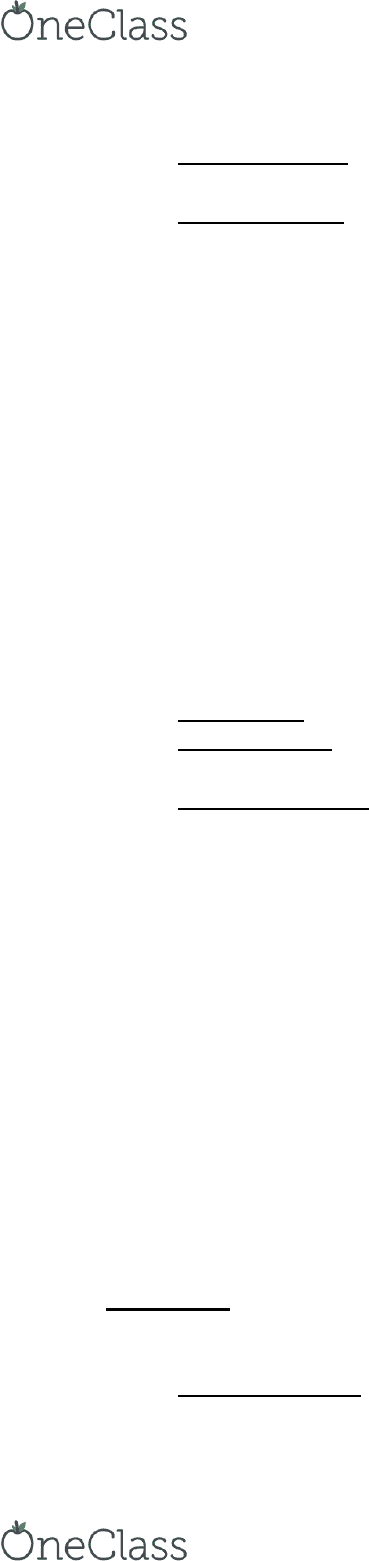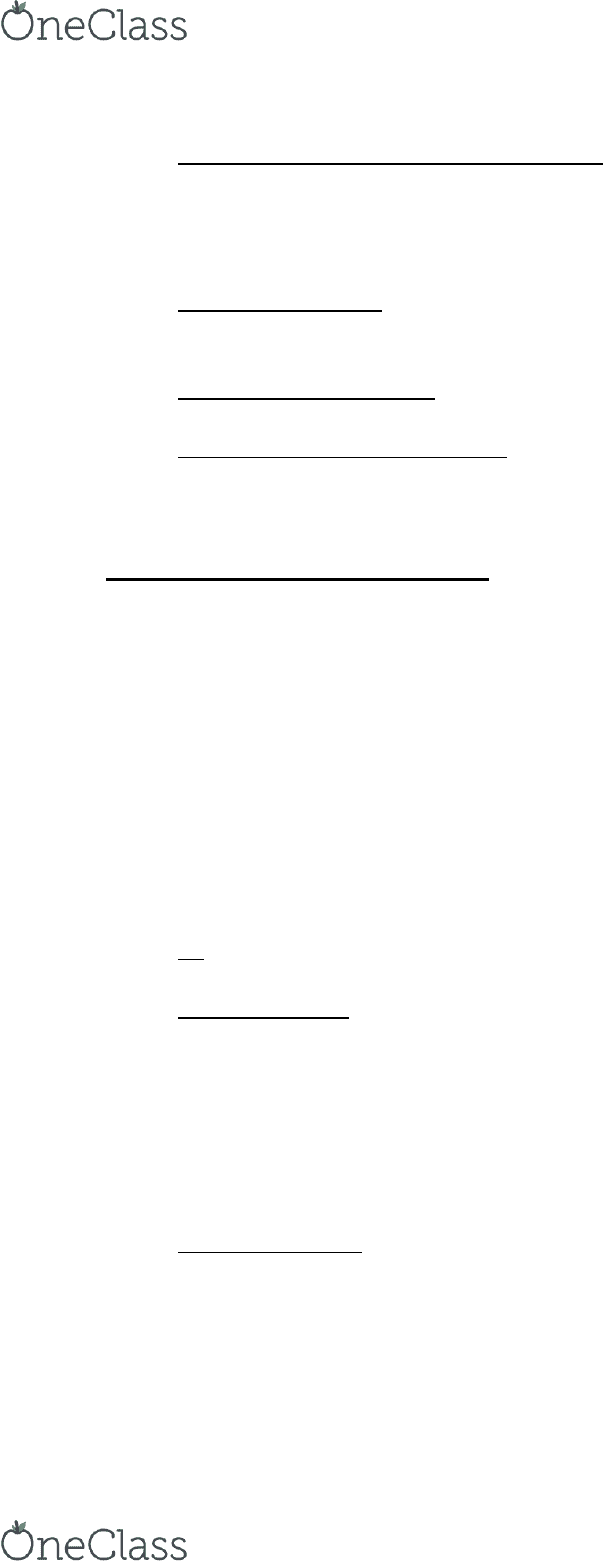# MATH 1007 Chapter Notes - Chapter 7-8 & 19-20: United States Congressional Apportionment, Highest Averages Method, Monotonicity Criterion

104 views11 pages
School
Department
Course
Professor

For unlimited access to Textbook Notes, a Class+ subscription is required.Samuel Mohebban Exam 2 Notes Math and Politics
Chapter 7- Hamilton’s Method
Apportionment- a method designed to round real numbers into whole numbers.
o Makig a fai shae of seats aodig to a state/outy populatio
Hamilton- a method od apportionment
o Deteie eah state’s fai shae (standard quota) by dividing its population by
the total population and multiplying this by the total number of seats to be
apportioned
o Then round these standard quotas either up to the next largest integer (upper
quota) or down to the next smallest integer (lower quota) in such a way that
(1) the correct number of seats have been doled out,
(2) the benefit of the the extra representative is doled out to the states in
decreasing order of the fractional part of their standard quotas
Fractional part is the decimal after a number (1.33= 33)
n- positive integer which stands for the number of states in the problem
o In the US n=50
h- the number of seats in the house of representatives
o In the U.S h=435
Pk- the population of the kth state
o Population of the first state is denoted P1 and the 17th state is denoted P17
p- the total population of all states
o p= p+p+….+pn
Census- refers to the numbers h, n, and p1,p2,pn collectively
o Output- is to be a list of n whole numbers that we donate by a1,a2,a3,an
ak- as the number of seats accorded to the kth state
o ak might equal 0 for some
a- is for apportionment
Apportionment Method- is a function whose domain is the set of all possible values of
h, n, and p1, p2, pn, where h and n are positive integers and the numbers p1, p2, pn are
positive real numbers, and whose input is a sequence of n nonnegative integers a1, a2,
an satisfying
o a+a+…+an=h
Represents the requirement that the total number of seats accorded to
the states must equal the prescribed house size exactly
o Goal is to make p/h as close to pn/an
o Fraction ak/pk (reciprocal) can be thought of as the measure of the strength in
voice in congress of a single citizen in the kth state
o For perfection, the apportionment number ak must be an integer
Standard quota- the real number qk= h(pk/p) for the kth state
o For Maryland, the standard quota = 435(5,307,886/281,424,177) = 8.2
Also can be shown in the form pk/(p/h)
o Standard Divisor- the denominator in this expression
Designated by the letter s= p/h
o Standard quota of a state- qk=pk/s (dividing its population by standard divisor)
find more resources at oneclass.com
find more resources at oneclass.com
Unlock document

This preview shows pages 1-3 of the document.
Unlock all 11 pages and 3 million more documents.

Already have an account? Log inSamuel Mohebban Exam 2 Notes Math and Politics
Standard divisor- represents the population of an ideal district
o Designated by the letter s= p/h
Standard Quota- represents the ideal number of seats to assign to that state
o The sum of all standard quotas = h
o Represented by qk=pk/s (dividing its population by standard divisor)
Two natural choices for ak is:
o Lower quota- the standard quota rounded down (8.2= 8)
o Upper quota- the standard quota rounded up (8.2= 9)
Ex. Estimates suggests that Montana will have a standard quota of 1.4.
Whether this will be rounded up to 2 or down to 1 is critically important
to the welfare of the Montanans. If Montana is allocated just one seat in
the House, the lone representative from this state will represent 905,316
people, making Montana the largest congressional district in the nation,
much larger than the average size of a district nationwide. On the other
hand, if Montana is allocated two seats, each congressional district in
Montana will contain 453,000 residents, far fewer than the national
average. The only choices for Montana are extremes, making the
residents either the most represented or the least represented
Americans.
Integer part- is the greatest integer less than or equal to the real number (3.141= 3)
Fractional Part- is the difference between that number and its integer part of a real
number (3.141= .141)
Hamilton Method- Assigns every state its lower quota. Then assigns the seats that
remain to the states, at most on per state, in decreasing order of the size of the
fractional parts of their standard quotas
o **example**
o assigns each state either its lower or upper quota
o Any method that does this is called the quota method
o Because the sum of the standard quotas = h, rounding all of them up would be
greater than h
o Hamilton argued that a state should be awarded a number of representatives
that is at least the integer part of its standard quota (the lower quota), and then
it should be considered for one additional seat based on the fractional part of
the standard quota
o Shows major flaws when applying the population, Alabama, and Oklahoma
paradoxes
o Violates population and house monotonicity criterion.
o Satisfies quota criterion (upper and lower quota rule)
Paradoxes
Hamilton method can behave weirdly in the face of changes to the house size, h, the
number of n states, or the populations p1,p2,pn of the states
Alabama Paradox- where a state loses a seat when the house size is increased
o **example**
find more resources at oneclass.com
find more resources at oneclass.com
Unlock document

This preview shows pages 1-3 of the document.
Unlock all 11 pages and 3 million more documents.

Already have an account? Log inSamuel Mohebban Exam 2 Notes Math and Politics
House monotone (monotonicity criterion)- criterion for apportionment that forbids the
Alabama paradox
o If an in increase in h, all other parameters remaining fixed, can never result in a
decrease in any ak
o Hailto’s ethod iolates this
Population Paradox- when one state gains in population while another loses, and yet in
the transition the first state loses a seat while the second gains a seat
o Hailto’s ethod iolates this
Population Monotonicity- a method that avoids the population paradox
o Hailto’s ethod iolates this
New states (Oklahoma) Paradox- the paradox showing the impact of the addition of
another state into the union
Chapter 8- Divisor Methods
h is fixed and given to us in advance, and our ob is to distribute exactly that many seats
In the U.S, the house size was not fixed in advance, but was determined by
congressional apportionment legislation
o First apportionment of the House was based on a census that allotted h=105
members. The number rose over decades, reaching its current value of h=435
If h is not predetermined, then another approach to apportionment is suggested
Jefferson proposed that instead of beginning with a fixed value of h, begin with a fixed
idea of how large a congressional district should be
When we have a fixed value of h, we use the standard divisor of s=p/h to represent this
quantity
When we do not have a fixed value of h, then we choose an arbitrary number d that we
regard as an appropriate size for a congressional district
d- is called the modified divisor
o Modified refers to the fact that the divisor is not the standard one
Modified divisor- Like the standard divisor s, we divide the modified divisor into the
population of each state
o Modified divisor for state k is pk/d
Represents the appropriate number of seats to assign to state k, if each
district is to have a population d
o Not always an integer
So Jefferson proposed that we round these numbers down to the next
whole number
Jefferson Method- choose a modified divisor d. Compute the modified quotas pk/d.
Round each of these numbers down to obtain ak. If a+a+…+a=h, the e hae
Jefferson apportionment. Otherwise, modify the divisor d and try again
o Usually d is smaller than s
o If d is too lage, the the total ue of seats, a+a+…+a ill e salle tha
h
o If d is too small, then the total ue of seats, a+a+…+a ill e lage tha h
find more resources at oneclass.com
find more resources at oneclass.com
Unlock document

This preview shows pages 1-3 of the document.
Unlock all 11 pages and 3 million more documents.

Already have an account? Log in

# Get access

Grade+
\$10 USD/m
Billed \$120 USD annually
Homework Help
Class Notes
Textbook Notes
40 Verified Answers
Study Guides
1 Booster Class
Class+
\$8 USD/m
Billed \$96 USD annually
Homework Help
Class Notes
Textbook Notes
30 Verified Answers
Study Guides
1 Booster Class